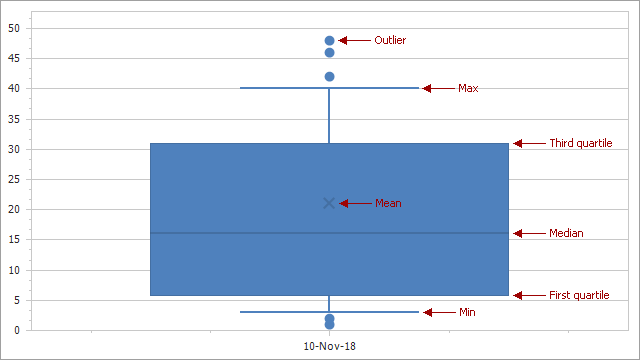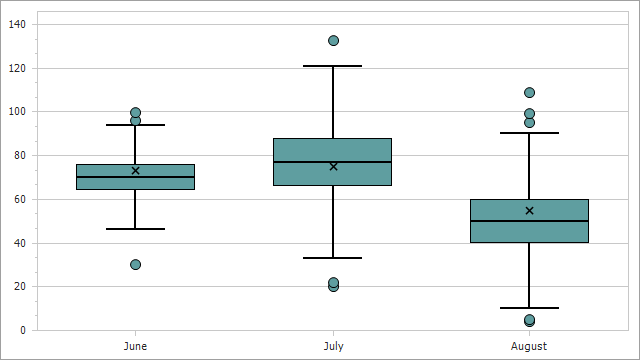# BoxPlotSeriesView Class

Represents a series view of the Box Plot type.

Namespace: DevExpress.XtraCharts

Assembly: DevExpress.XtraCharts.v22.2.dll

NuGet Package: DevExpress.Charts

## Declaration

``````public class BoxPlotSeriesView :
SeriesViewColorEachSupportBase,
ISideBySideBarSeriesView,
IBarSeriesView,
ISupportBorderVisibility,
IGeometryHolder,
ISupportTransparency``````

## Remarks

The following image shows a Box Plot chart and its elements:To create a Box Plot point, you should specify the following parameters:

• Min
• Max
• First Quartile
• Third Quartile
• Median

The following parameters are optional:

• Mean
• Outliers

Refer to the Providing Data section for more about different approaches on how to populate a series with points.

The BoxPlotSeriesView class introduces the options that allow you to customize the appearance of Box Plot chart elements:

Use the BoxDistance and BoxDistanceFixed properties to change a gap between points of different series in the same chart.

To change series animation-related settings, use the Animation property.

## Example

This example shows how to create a Box Plot chart.``````using DevExpress.XtraCharts;
using System;
using System.Collections.Generic;
using System.Drawing;
using System.Windows.Forms;

namespace BoxPlotChart {
public partial class Form1 : Form {
public Form1() {
InitializeComponent();
}
private void Form1_Load(object sender, EventArgs e) {
Series series = new Series("Box Plot", ViewType.BoxPlot);
series.DataSource = DataPoint.GetDataPoints();
series.SetBoxPlotDataMembersWithOutliers("Argument", "Min", "Quartile1", "Median", "Quartile3", "Max", "Mean", "Outliers");

BoxPlotSeriesView view = series.View as BoxPlotSeriesView;
view.EqualBoxWidth = true;
view.CapWidthPercentage = 50;
view.MeanAndMedianColor = Color.Black;
view.MeanMarkerKind = MarkerKind.ThinCross;
view.MeanMarkerSize = 10;
view.Border.Color = Color.Black;
view.Border.Thickness = 1;
view.Border.Visibility = DevExpress.Utils.DefaultBoolean.True;

XYDiagram diagram = chartControl1.Diagram as XYDiagram;
diagram.AxisX.Tickmarks.MinorVisible = false;
}
}
public class DataPoint {
public string Argument { get; set; }
public double Min { get; set; }
public double Max { get; set; }
public double Quartile1 { get; set; }
public double Quartile3 { get; set; }
public double Median { get; set; }
public double Mean { get; set; }
public double[] Outliers { get; set; }
public DataPoint(string argument, double min, double max, double q1, double q3, double median, double mean, double[] outliers) {
this.Argument = argument;
this.Min = min;
this.Max = max;
this.Quartile1 = q1;
this.Quartile3 = q3;
this.Median = median;
this.Mean = mean;
this.Outliers = outliers;
}
public static List<DataPoint> GetDataPoints() {
List<DataPoint> data = new List<DataPoint> {
new DataPoint("June", 46, 94, 64, 76, 70, 73, new double[]{ 30, 96.3, 99.56 }),
new DataPoint("July", 33, 121, 66, 88, 77, 75, new double[]{ 20, 22, 132.7 }),
new DataPoint("August", 10, 90, 40, 60, 50, 55, new double[]{ 4, 5, 95.4, 99.3, 109 })
};
return data;
}
}
}
``````